Next: Logistic Map Up: Theoretical Analysis Previous: Signed Binary Stream Multiplication

## Division

The algorithms described so far all have all had lookaheads of the form (n+c). Here we examine the division algorithm, whose lookahead behaviour is worse than this.

We first observe that there is no danger of the size of the dyadic digits swelling as we only generate one of seven possible digits at each step and that these are not used in other operations except in conversion back into signed binary digits.

Suppose we are computing the division x/y. In order to examine the lookahead we concentrate on the numerator x. It is possible to see from the way that the denominator is used that the lookahead in the denominator stream will always be less than or equal to the lookahead in the numerator stream.

The algorithm first removes all ,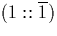, and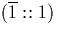combinations from the start of the numerator and adds an appropriate value to the exponent to leave the number represented unchanged. This is a fixed overhead and is not expensive except in as much as the numerator may itself be an expression which must be calculated digit by digit. The algorithm now proceeds by examining cases. The conversion of the dyadic result back into signed binary requires (n+1) dyadic digits of input to obtain n of output. We examine each case in turn and show how it affects the lookahead and branching.

 Digits of x Digits of (x-y) Digits required to obtain n digits of numerator of the recursive call (in general). Branching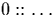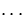1c|n+1 None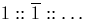1c|n+1 None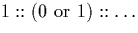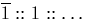1c|n+3 1 average1c|n+3 1 average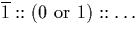1c|n+3 1 average1c|n+3 1 average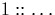1c|n+4 2 averages1c|n+1 None1c|n+3 1 average1c|n+3 1 average1c|n+3 1 average1c|n+3 1 average1c|n+4 2 averages

We now use these observations to work out how the lookahead for division behaves. To obtain one digit of output will require at most four digits of the numerator. In order to obtain two digits will require no more than four digits of the numerator of the recursive call, or eight digits of the original input. It is clear that the lookahead is at most (4n + c), although for most cases the lookahead at each step is less than this, so for an average operation in which each case is equally likely a lookahead of 3n + c would be more likely. The lower bound is (n + c). The algorithm branches. For n digits of output we would expect roughly n concurrent average operations to be required.Next: Logistic Map Up: Theoretical Analysis Previous: Signed Binary Stream Multiplication
Martin Escardo
5/11/2000